# Team:Oxford/Model/Design

## Part I: Dynamic Expression vs. Constitutive Expression of Endolysin

### Assumptions

• Each L. reuteri cell produces the theoretically predicted concentration of endolysin.
• When endolysin production is induced in the dynamic expression system, all cells begin synthesis simultaneously.

### Kinetic Rates

#### a) Concentration of endolysin produced per L. reuteri cell required for lysis of C. difficile cells estimated from experimental killing assay data of surrogate target bacterium B. subtilis (further detailed in the Supplementary Materials page.)

Endolysin Concentration Produced per L. reuteri cell /M CFU -1
0.00465

#### b) Stress on L. reuteri growth rate due to constitutive expression of CD27L endolysin:

Type of L. reuteri Doubling time /hr Growth Rate (ln 2/Doubling Time) /hr -1
Wild Type 0.548 1.26
Consitutively Expressing CD27L Endolysin 9.08 0.76

#### Species Key: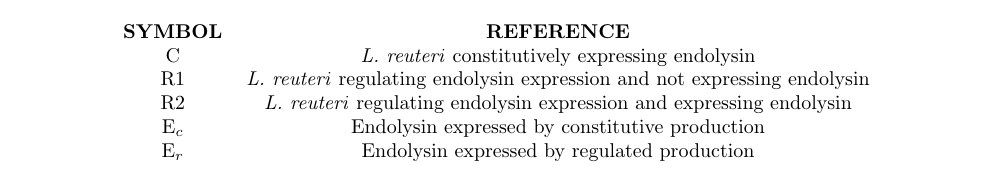Process Modelled Differential Equation 1 Explanation 1 Differential Equation 2 Explanation 2
Logistic Growth Model of L. reuteri - Constitutive Expression $$\frac {dC}{dt} = r_{stressed} \times C \times (1- \frac {C}{N_{lr}})$$ Endolysin is being expressed constitutively. N/A N/A
Logistic Growth Model of L. reuteri - Dynamic Expression $$\frac {dR_{1}}{dt} = r_{wildtype} \times R \times (1- \frac {R}{N_{lr}})$$ If endolysin is not being expressed. $$\frac {dR_{2}}{dt} = r_{stressed} \times R \times (1- \frac {R}{N_{lr}})$$ If endolysin is being expressed.
Linear Expression of Endolysin $$\frac {dEc}{dt} = k \times C$$ For constitutive expression by L. reuteri $$\frac {dEr}{dt} = k \times R$$ For dynamic expression by L. reuteri

### Results

#### Legend

Colour of Graph Line Explanation
Dotted L. reuteri constitutively expressing endolysin.
Green Endolysin yield increases with the time of induction of endolysin expression
Blue Endolysin yield decreases with the time of induction of endolysin expression
Red Endolysin yield of note is below endolysin yield from constitutive expression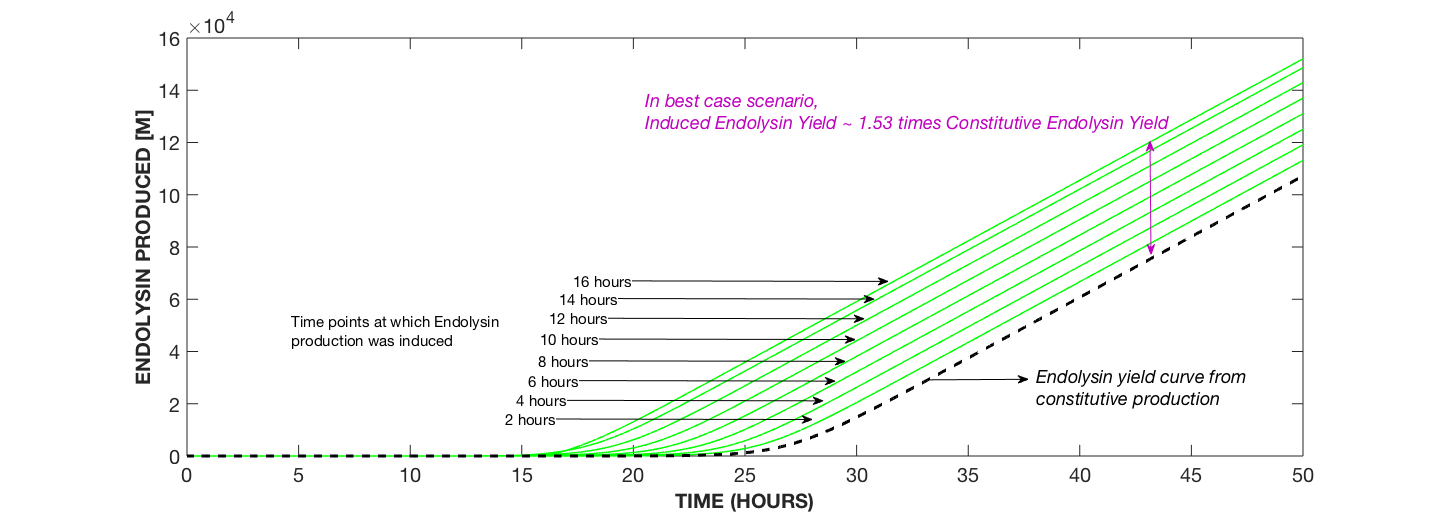Fig. 1a compares the yield of endolysin from constitutive expression to that from dynamic expression whereby yield increases with increase in the time of induction.

#### After the 16 hour time point, endolysin yields decrease with increase in the induction time. This is seen in Figure 1b below.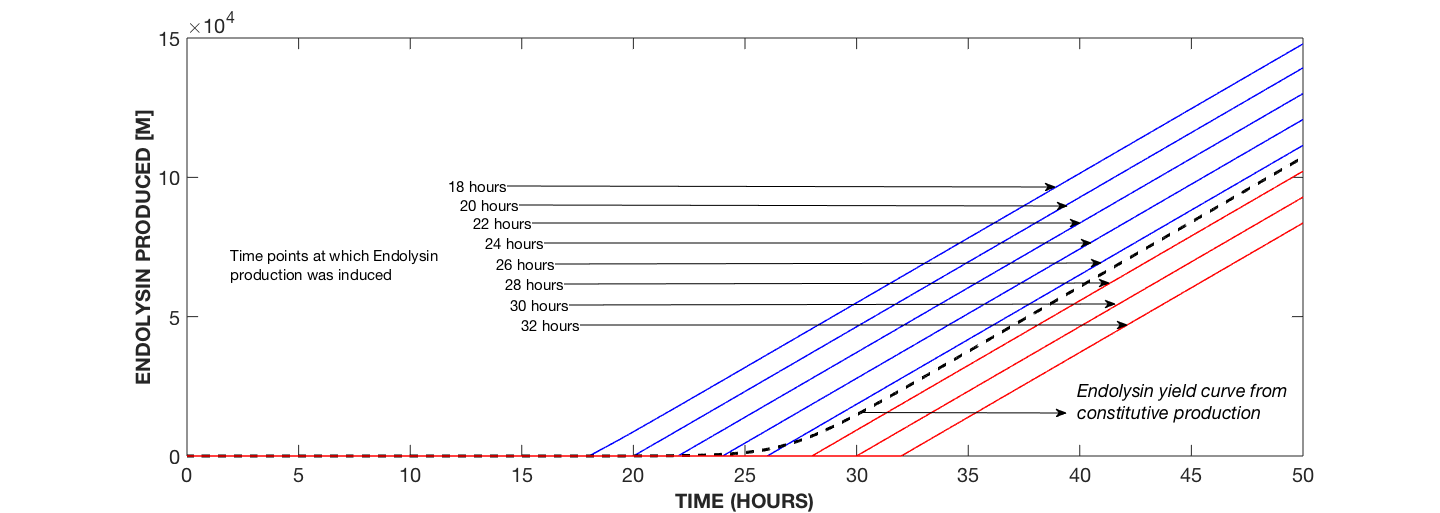Fig. 1b compares the yield of endolysin from constitutive expression to that from dynamic expression whereby yield decreases with increase in the time of induction.

#### To analyse the survivability of L. reuteri dynamically expressing endolysin to L. reuteri constitutively expressing endolysin, we plotted the growth curves corresponding to the endolysin yields above. The results are shown in Figure 2 below.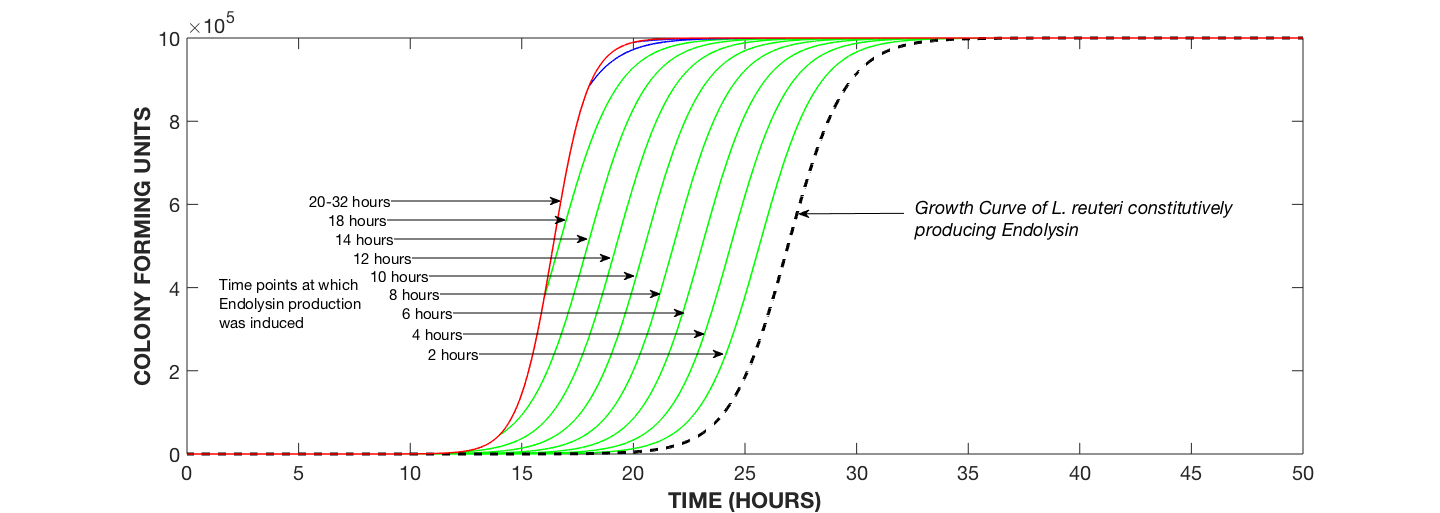Fig 2: Growth Curves of L. reuteri Undergoing Endolysin Expression with Induction Times ranging from 2 hrs to 32 hrs.

### Discussion

#### From the results above, regulated production has advantages over constitutive production in:

1. Endolysin Yield
2. #### If endolysin expression is induced before 18 hours, dynamic endolysin expression results in greater endolysin yields from L. reuteri than constitutive expression.

3. Survivability of L. reuteri

## Part II: Mathematical Model of Two-Component Regulatory System (TCS)

### Regulatory System Requirements

#### For optimal function of our overall system, the regulatory system must meet certain requirements:

• Endolysin expression should be triggered when the surrounding population of C. difficile crosses a certain threshold.
• The regulatory system must be robust to temporary spikes of external signal(s).
• Endolysin synthesis should be speedy and produce sufficient amounts of endolysin so that the surrounding population of C. difficile diminishes before toxin synthesis occurs significantly.

### Regulatory System Primer

#### C. difficile uses a two-component system (TCS) to detect its signalling molecule (AIP). As seen below, when the AIP molecule binds to its AgrC receptor, it triggers a phosphorylation cascade: the receptor first autophosphorylates; this causes the phosphorylation of AgrA protein which then dimerises. The dimerised AgrA protein acts as the specific transcription factor for the expression of CD27L endolysin.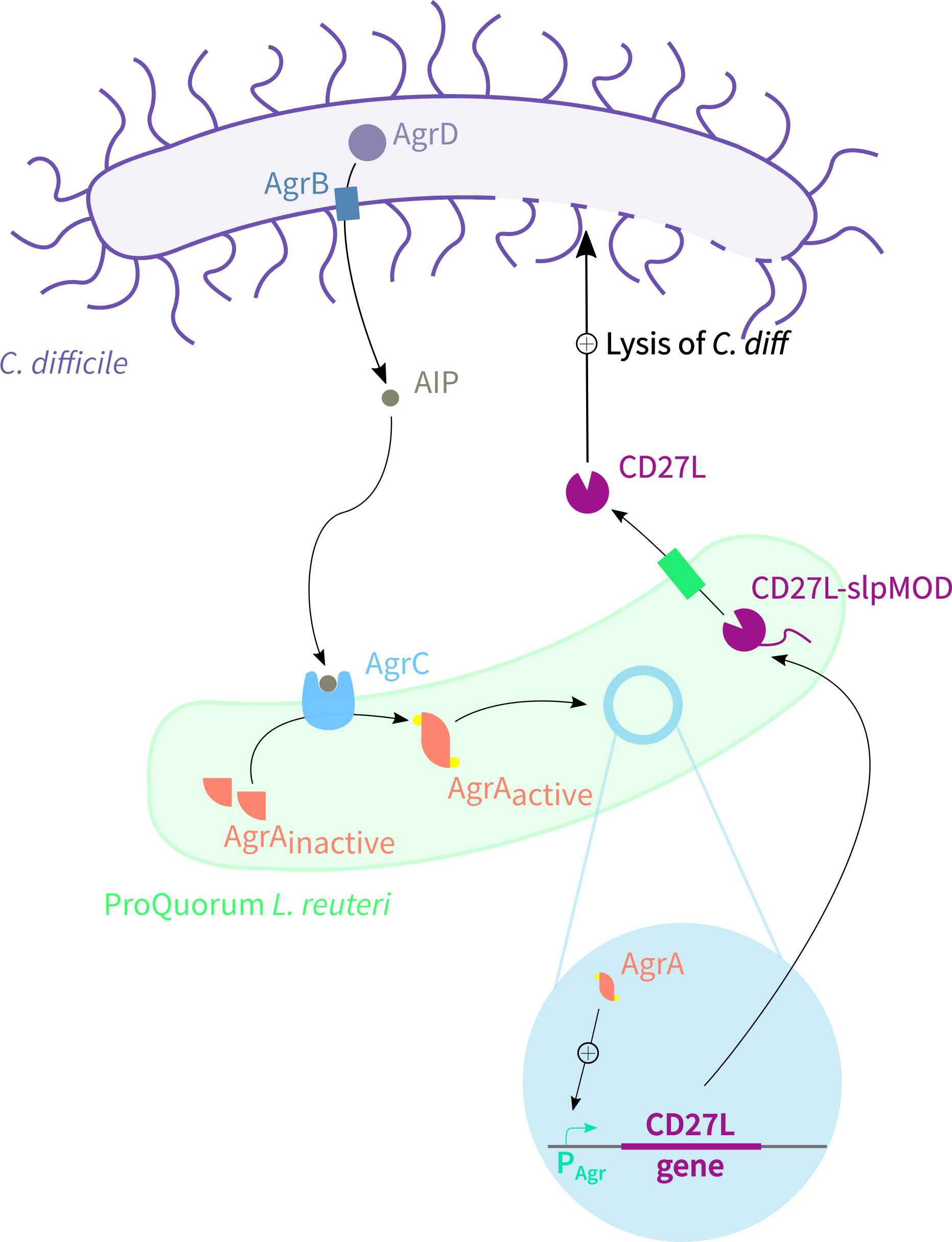### Assumptions

1. The modelled environment is uniformly mixed, hence spatial dependencies can be ignored.
2. Dephosphorylation of AgrA protein occurs via housekeeping phosphatases.
3. There is negligible decrease in the concentration of dimerised AgrA protein due to promoter binding as binding of one molecule of dimerised AgrA protein to the promoter is sufficient to trigger endolysin synthesis.
4. Timescale of degradation of all proteins is approximated to be equal as in Jabbari et al. (2009)1. Consequently, rate of degradation is the same.

#### Species Key: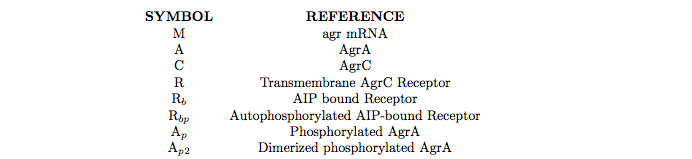### Transcription

Kinetic Rate Equation Kinetic Rate Constant Kinetic Rate Constant Value Modelled
$$[NULL] \to M$$ $$m$$ $$8.33 \ M cell^{-1} \ minute^{-1}$$

### Translation + Membrane Insertion of Cytoplasmic AgrC Receptor

Kinetic Rate Equation Kinetic Rate Constant Kinetic Rate Constant Value Modelled
$$M \to M + A$$ $$K_a$$ $$8.33 \times 10^{-8} \ minutes^{-1}$$
$$M \to M + C$$ $$K_c$$ $$8.33 \times 10^{-6} \ minutes^{-1}$$
$$C \to R$$ $$\alpha_C$$ $$0.0556 \ minutes^{-1}$$

### AIP Binding to Transmembrane AgrC Receptor

Kinetic Rate Equation Kinetic Rate Constant Kinetic Rate Constant Value Modelled Inverse Kinetic Rate Constant Inverse Kinetic Rate Constant Value Modelled
$$S + R \leftrightarrow R_b$$ $$k_{bind}$$ $$1.67 \times 10^{-7} \ (M \times minute)^{-1}$$ $$k_{ibind}$$ $$1.167 \ minutes^{-1}$$

### Autophosphorylation of AgrC Receptor

Kinetic Rate Equation Kinetic Rate Constant Kinetic Rate Constant Value Modelled Inverse Kinetic Rate Constant Inverse Kinetic Rate Constant Value Modelled
$$R_b \leftrightarrow R_{bp}$$ $$k_{autop}$$ $$6.3133 \ minutes^{-1}$$Ref. 3 $$k_{iautop}$$ $$10^{-2} \ minute^{-1}$$

### Phosphotransfer to AgrA Protein

Kinetic Rate Equation Kinetic Rate Constant Kinetic Rate Constant Value Modelled Inverse Kinetic Rate Constant Inverse Kinetic Rate Constant Value Modelled
$$R_{b_p} + A \leftrightarrow R_b + A_p$$ $$k_{phosph}$$ $$5 \times 10^5 \ minutes^{-1}$$ $$k_{dphosph}$$ $$0.1777 \ minutes^{-1}$$Ref. 4

### Dimerisation of AgrA Protein

Kinetic Rate Equation Kinetic Rate Constant Kinetic Rate Constant Value Modelled Inverse Kinetic Rate Constant Inverse Kinetic Rate Constant Value Modelled
$$2A_p \leftrightarrow A_{p_2}$$ $$\phi_d$$ $$3.33 \times 10^{8} \ (M \times minute)^{-1}$$ $$\phi_m$$ $$0.0167 \ minute^{-1}$$

Kinetic Rate Equation Kinetic Rate Constant Kinetic Rate Constant Value Modelled
$$X \to NULL \ (where X=M, A, C, R, Rb, Rbp, S, Ap, Ap_2)$$ $$\delta_X$$ $$0.05566 \ minutes^{-1}$$Ref. 2

#### Differential Equations

Concentration Modelled Differential Equation Process Modelled
mRNA of AgrC Receptor & AgrA Protein $$\frac {dM}{dt} = m - \delta_{M} \times M$$ Transcription of AgrC & AgrA Genes + Degradation of mRNA
Deactivated AgrA Protein $$\frac {dA}{dt} = K_a \times M - \delta_A \times A - k_{phosph} \times R_{bp} \times A + k_{dphosph} \times R_b \times A_p$$ Translation of AgrA Protein + Degradation of AgrA Protein + Phosphorylation/Dephosphorylation of AgrA
Cytoplasmic AgrC Receptor $$\frac {dC}{dt} = K_a \times M - \alpha_C \times C - \delta_C \times C$$ Translation of AgrC Receptor + Membrane Insertion of AgrC Receptor + Degradation of AgrC Receptor
Unbound AgrC Receptor $$\frac {dR}{dt} = \alpha_C \times C - \delta_R \times R + k_{ibind} \times R_b - k_{bind} \times R \times S$$ Membrane Insertion of AgrC Receptor + Degradation of Inserted AgrC Receptor + Binding/Unbinding of AgrC Receptor
Bound AgrC Receptor $$\frac {dR_b}{dt} = k_{bind} \times S \times R - k_{ibind} \times R_b - \delta_{R_b} \times R_b + k_{iauto} \times R_{bp} - k_{autop} \times R_b + k_{phosph} \times R_{b_p} \times A - k_{dphosph} \times R_b \times A_p$$ Ligand Binding/Unbinding of AgrC Receptor + Degradation of Bound AgrC Receptor + Autophosphorylation/De-autophosphorylation + Phosphotransfer/Dephosphorylation of AgrC Receptor
Phosphorylated AgrC Receptor $$\frac {dR_{b_p}}{dt} = k_{autop} \times R_b - k_{iauto} \times R_{b_p} - k_{phosph} \times R_{b_p} \times A + k_{dphosph} \times R_b \times A_p - \delta_{Rb_p} \times R_{b_p}$$ Autophoshorylation/Auto-dephosphorylation of AgrC Receptor + Phosphotransfer/Dephosphorylation to AgrA Protein + Degradation of Phosphorylated AgrC Receptor
AgrA Protein $$\frac {dA_p}{dt} = k_{phosph} \times R_{bp} \times A - k_{dphosph} \times R_b \times A_p - 2 \times \phi_d \times A_{p}^2 + \phi_m \times A_{p_2} - \delta_{A_p} \times A_p$$ Phosphotransfer/Dephosphorylation of AgrA Protein + Dimerisation/Monomerisation + Degradation of AgrA Protein
Dimerised AgrA Protein $$\frac {dA_{p_2}}{dt} = \phi_d \times A_{p}^2 - 0.5 \times \phi_m \times A_{p_2} - \delta_{Ap_2} \times A_{p_2}$$ Dimerisation/Monomerisation of AgrA Protein + Degradation of Dimerised AgrA Protein

### Upregulation of Cells

#### The particular parameters are explained in the table below.

Parameter Explanation
b Binding Rate of Transcription Factor
u Unbinding Rate of Transcription Factor
Ap Population-wide Concentration of Transcription Factor in the S. aureus Quorum Sensing System
N Number of Cells

### Results

#### To analyse the key features of the two-component regulatory system within each cell, we ran cellular-scale simulations in which the cell is exposed to various signal concentrations and patterns.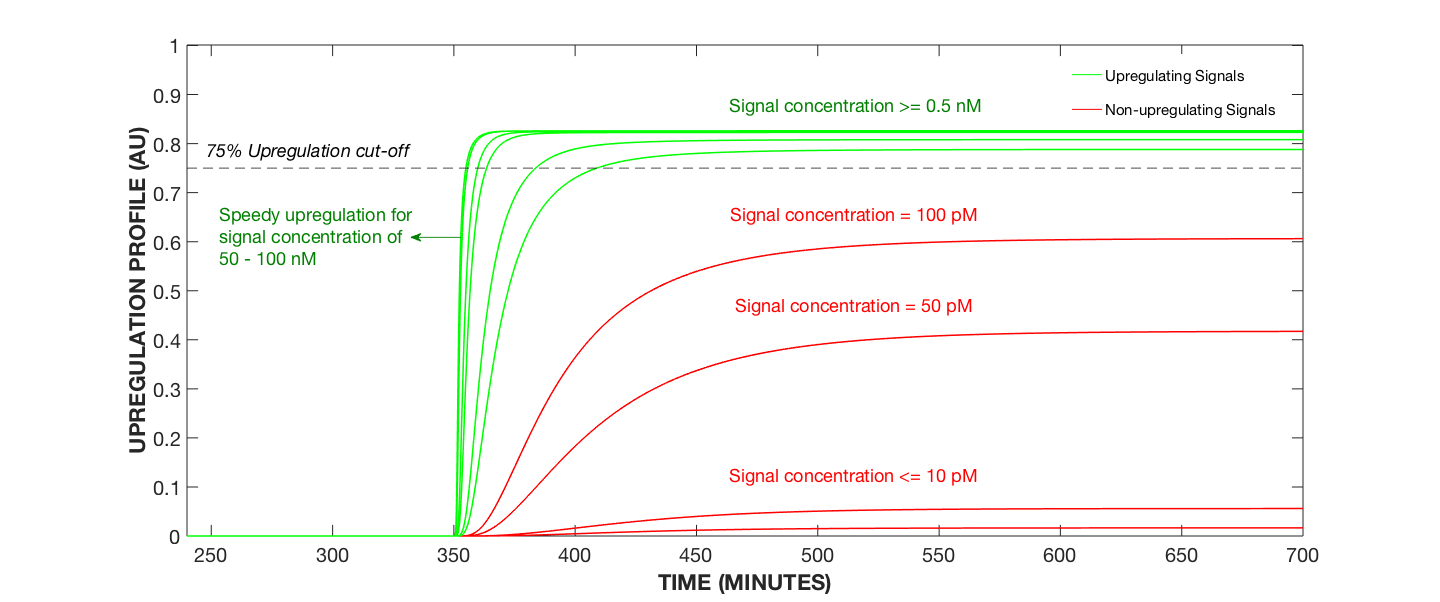Figure 1. Upregulation Profile for Varying AIP Concentrations

#### To observe how the concentration of the transcription factor varies during upregulation and downregulation, we ran a simulation where an AIP concentration of 1nM was inserted at 350 hours and taken away at 650 hours.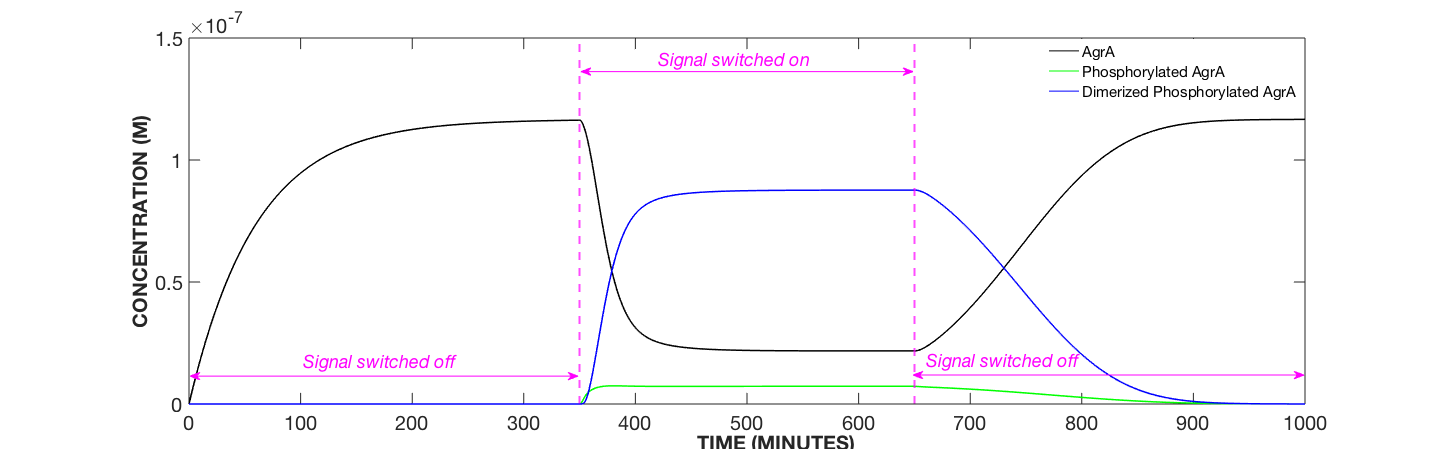Figure 2. Transcription Factor Dynamics, AIP Concentration Modulated

#### The dynamics of AgrA caused by the modulation of AIP concentration is reflected in the upregulation profile of the cell as depicted in Figure 3 below.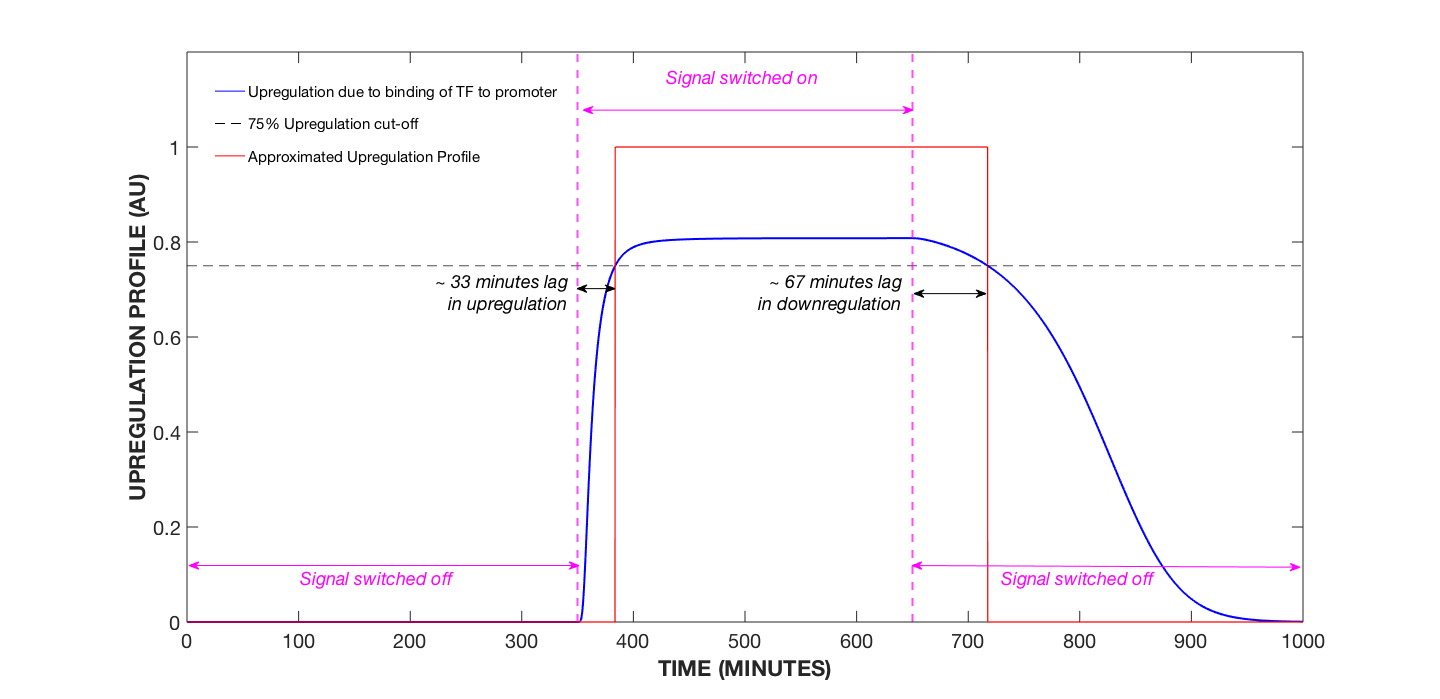Figure 3. Upregulation Profile of Cell, AIP Concentration Modulated

#### For further insight into the delay in upregulation of the cell, we plotted the maximum upregulation state of the cell for different signal durations given 1 nM AIP signal concentrations.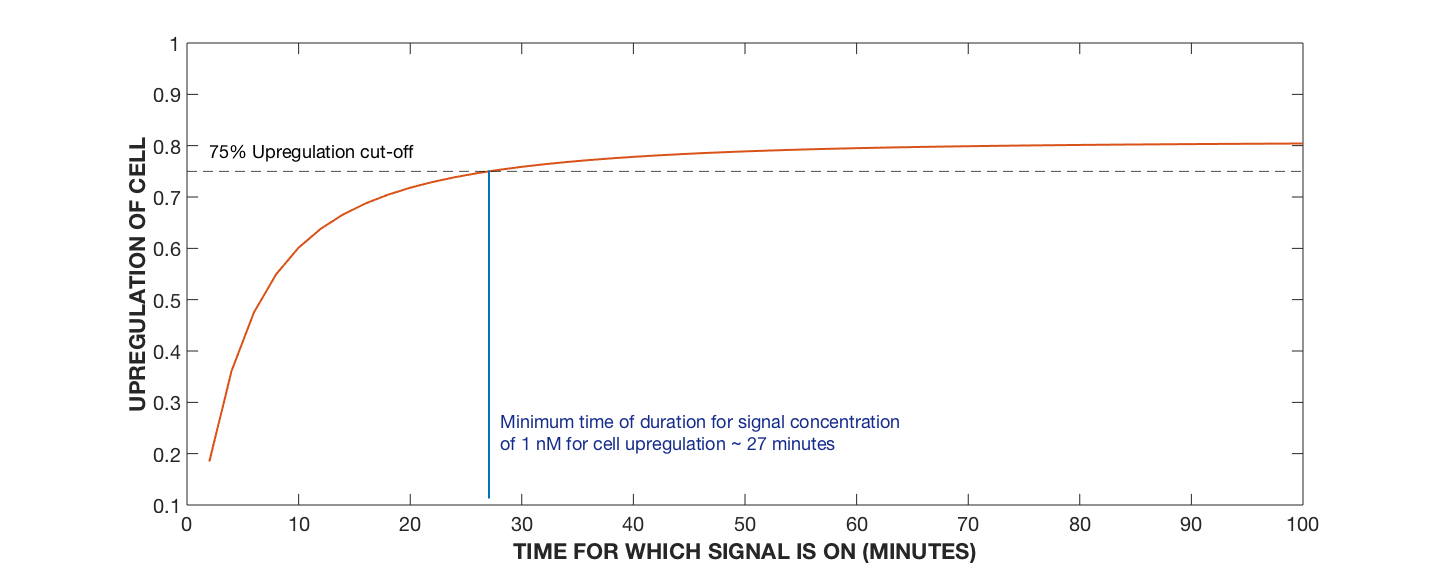Figure 4. Upregulation Profile of Cell, Varying AIP Signalling Time

#### When running the same simulation for different signal concentrations, we obtain the following graph: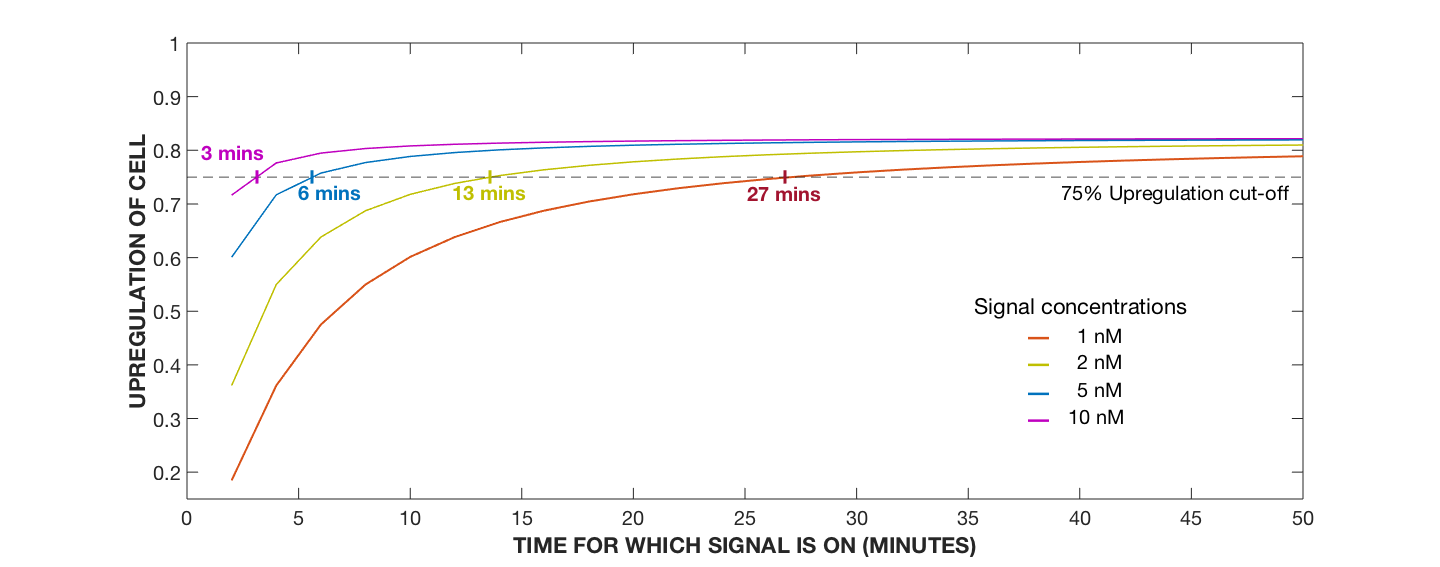Figure 5. Upregulation Profile of Cell, Varying AIP Signalling Time with Varying AIP Concentrations

#### As seen in Figure 6 below, the cell was ultimately upregulated by AIP signal concentrations in the picomolar range: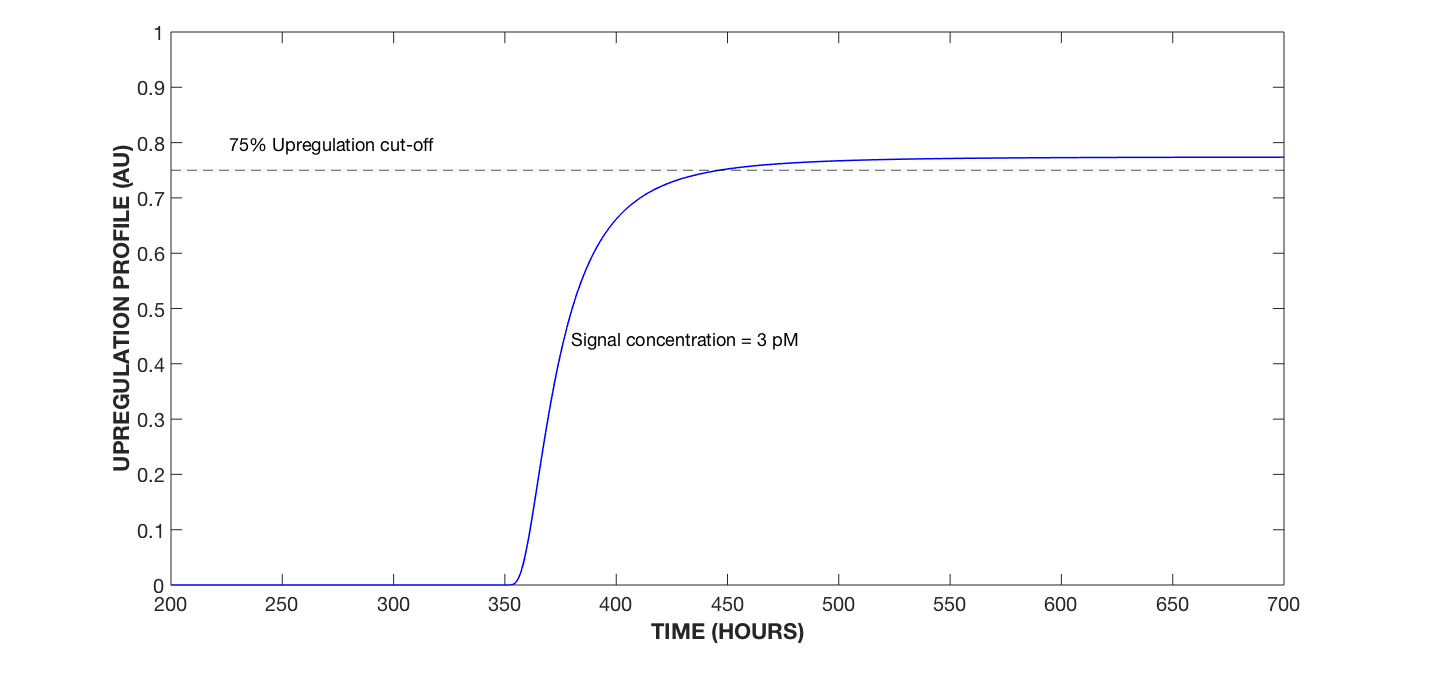Figure 6. Upregulation Profile of Cell, 3 pM AIP Signal Concentration

### Discussion

#### The two-component regulatory system employed by C. difficile has been confirmed via our model to meet the following design requirements:

• Endolysin expression is triggered only after the concentration of AIP crosses a certain threshold - a valid indicator of C. difficile population density.
• Endolysin expression is robust to sudden and short-lived spikes in AIP concentration due to its intrinsic delay in response.

## References

# Reference
1 Jabbari, Sara, et al. “Mathematical Modelling of the Agr Operon in Staphylococcus Aureus.” Journal of Mathematical Biology, vol. 61, no. 1, 2009, pp. 17–54., doi:10.1007/s00285-009-0291-6.
2 Wright, J. S., et al. “Transient Interference with Staphylococcal Quorum Sensing Blocks Abscess Formation.” Proceedings of the National Academy of Sciences, vol. 102, no. 5, 2005, pp. 1691–1696., doi:10.1073/pnas.0407661102.
3 Srivastava, S. K., et al. “Influence of the AgrC-AgrA Complex on the Response Time of Staphylococcus Aureus Quorum Sensing.” Journal of Bacteriology, vol. 196, no. 15, 2014, pp. 2876–2888., doi:10.1128/jb.01530-14.
4 Wang, Boyuan, and Tom W. Muir. “Regulation of Virulence in Staphylococcus Aureus : Molecular Mechanisms and Remaining Puzzles.” Cell Chemical Biology, vol. 23, no. 2, 2016, pp. 214–224., doi:10.1016/j.chembiol.2016.01.004.